# 13.17 The vector type

append(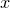)
The append() method appends the numberto a vector and returns the new vector.

extend()
The extend() method appends the members of a list or vectorto the operand and returns the new vector.

filter(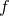)
The filter() method takes a pointer to a function of one argument,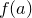. It calls the function for every element of the vector, and returns a new vector of those elements for whichtests true.

insert()
The insert() method inserts the numberinto a vector at position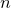, and returns the new vector.

len()
The len() method returns the number of elements in a vector.

list()
The list() method returns the elements in a vector as a list.

map()
The map() method takes a pointer to a function of one argument,. It calls the function for every element of the vector, and returns a vector of the results.

norm()
The norm() method returns the quadrature sum of the elements in a vector.

reduce()
The reduce() method takes a pointer to a function of two arguments. It first calls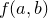on the first two elements of the vector, and then continues through the vector callingon the result and the next item in the vector. The final result is returned.

reverse()
The reverse() method reverses the order of the elements of a vector, and returns the new vector.

sort()
The sort() method sorts the elements of a vector into ascending order, and returns the new vector.